# Iterator Invalidation in C++

## Iterator Invalidation in C++ with Examples:

In this article, we will study Iterator Invalidation in C++ with Examples, and also, we will discuss the different rules of iterator invalidation. Please read our previous article, where we discussed Iterators in C++ with Examples. So first let us understand Iterator Invalidation in C++.

##### Iterator Invalidation in C++:

When there are changes in the content of the container and by changes, we mean when we insert or delete some elements to the container and that container is currently using iterators then these iterators may become invalidated. This may or may not be invalidated depending on the container implementation. So, they will not cause runtime errors but the iterator is no longer guaranteed to access the same element it was referring to before the update happened.

There are some well-documented rules for our different iterator invalidations and we will look at that. So first let’s look at an example.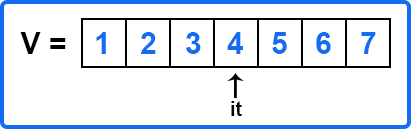Let us say we have a vector v and we have an iterator ‘it’ pointing at the fourth element. Then we have called insert on this and we have inserted 100 before 4 so now it becomes,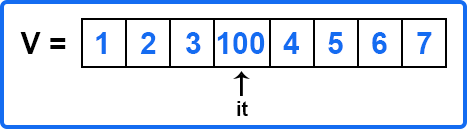But ‘it’ may still be pointing to 100 locations. So, before the update, it was pointing to a location that contains 4 and now it is pointing to a location that contains 100. So, you may or may not see this behavior. Let us say in some cases, here 1, 2, and 3 are not invalidated but the element place where we inserted it, and thereafter, it gets invalidated. but in the case of some other containers like list or even the forward list, in both cases, if we insert, if there is an iterator pointing to this location having x and we insert some value here then still it will be pointing to x only.

Because it is actually pointing to the object’s location. These may not be contiguous and it is actually pointing to the actual location of that element so that is not changed. We have just inserted some new nodes in between. So, in that case ‘it’ will not be invalidated. Let us first look at an example where we will show an iterator invalidation in the case of vectors. Then we will look at what are all the different rules and different containers.

##### Example to Understand Iterator Invalidation in C++
```#include <iostream>
#include <vector>
using namespace std;
int main()
{
vector<int> v = {1, 2, 3, 4, 5, 6, 7};
auto it = v.begin() + 4;  // 5

cout << "it => " << *it << endl;
cout << "it - begin=> " << it - v.begin()<< endl;
return 0;
}
```
###### Output: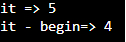In the above program, we have a vector v that contains 7 elements. Then we have an iterator that is pointing to the 5 elements of v. Then we have dereferenced the ‘it’ iterator. Now let us insert something in vector v.

```#include <iostream>
#include <vector>
using namespace std;
int main()
{
vector<int> v = {1, 2, 3, 4, 5, 6, 7};
auto it = v.begin() + 4;  // 5

cout << "it => " << *it << endl;
cout << "it - begin=> " << it - v.begin()<< endl;

v.insert(it, 100);
cout << "it => " << *it << endl;
cout << "it - begin=> " << it - v.begin()<< endl;

return 0;
}
```
###### Output: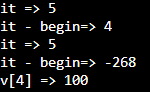Here we have inserted 100 at the 4th location in the vector v. Not it-begin shows a negative value. So why it is showing a negative value? Here ‘it’ is no longer valid. So, we can validate it. We have to change v.insert(it, 100) to it = v.insert(it, 100). Now the ‘it’ iterator will be updated. It will be valid and it should return 4. So, the code is as follows:

```#include <iostream>
#include <vector>
using namespace std;
int main()
{
vector<int> v = {1, 2, 3, 4, 5, 6, 7};
auto it = v.begin() + 4;  // 5

cout << "it => " << *it << endl;
cout << "it - begin=> " << it - v.begin()<< endl;

it = v.insert(it, 100); // {1, 2, 3, 100, 4, 5, 6, 7};
cout << "it => " << *it << endl;
cout << "it - begin=> " << it - v.begin()<< endl;
cout << "v => " << v << endl;

return 0;
}
```
###### Output: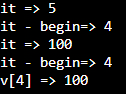When you have to insert or something like this then you update the iterator as well. Maybe you have a requirement where you want to insert something based on the condition. Let us say you are iterating through this vector and as soon as you find a value let’s say 10 then you insert before that. It may be a part of your requirement. In that case, you may write code like this and your code will become invalid if you are doing further operations on iterators. So, make sure that you update this or validate this iterator.

We hope this gave you some sense of iterator invalidation and how to prevent it. So, this was a vector. This would not be a problem with lists or forward lists or even maps and other containers. Let us see the complete rules.

##### Iterator Invalidation Rules in C++: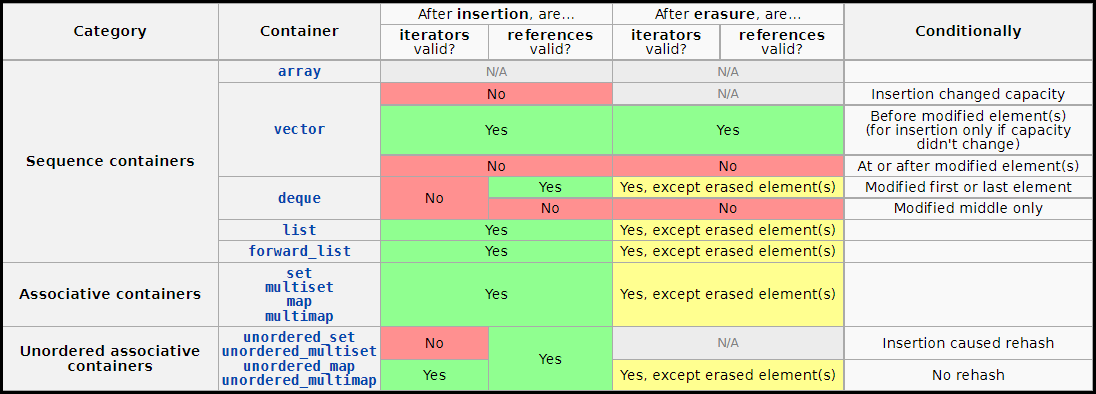You can refer to the above site. There will be a section for iterator invalidation. For example, if the container is an array, then in this case nothing is applicable since it’s a fixed-size container and you are not allowed to add or insert new elements. You are just allowed to update the value. Then we have a vector. After insertion and after eraser so there are two kinds of updates. You can insert some value or delete some value. So, you can go through this complete list.